# A chemist titrates 190.0 mL of a 0.4427 Macetic acid (HCH,CO, solution with 0.2382 M NaOH...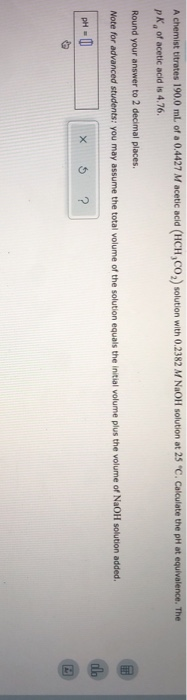A chemist titrates 190.0 mL of a 0.4427 Macetic acid (HCH,CO, solution with 0.2382 M NaOH solution at 25 "C. Calculate the pH at equivalence. The pk of acetic acid is 4.76. Round your answer to 2 decimal places. Note for advanced students: you may assume the total volume of the solution equals the initial volume plus the volume of NaOH solution added. pH = 0 x 5 ?

use:

pKa = -log Ka

4.76 = -log Ka

Ka = 1.738*10^-5

find the volume of NaOH used to reach equivalence point

M(HCH3CO2)*V(HCH3CO2) =M(NaOH)*V(NaOH)

0.4427 M *190.0 mL = 0.2382M *V(NaOH)

V(NaOH) = 353.1192 mL

Given:

M(HCH3CO2) = 0.4427 M

V(HCH3CO2) = 190 mL

M(NaOH) = 0.2382 M

V(NaOH) = 353.1192 mL

mol(HCH3CO2) = M(HCH3CO2) * V(HCH3CO2)

mol(HCH3CO2) = 0.4427 M * 190 mL = 84.113 mmol

mol(NaOH) = M(NaOH) * V(NaOH)

mol(NaOH) = 0.2382 M * 353.1192 mL = 84.113 mmol

We have:

mol(HCH3CO2) = 84.113 mmol

mol(NaOH) = 84.113 mmol

84.113 mmol of both will react to form CH3CO2- and H2O

CH3CO2- here is strong base

CH3CO2- formed = 84.113 mmol

Volume of Solution = 190 + 353.1192 = 543.1192 mL

Kb of CH3CO2- = Kw/Ka = 1*10^-14/1.738*10^-5 = 5.754*10^-10

concentration ofCH3CO2-,c = 84.113 mmol/543.1192 mL = 0.1549M

CH3CO2- dissociates as

CH3CO2- + H2O -----> HCH3CO2 + OH-

0.1549 0 0

0.1549-x x x

Kb = [HCH3CO2][OH-]/[CH3CO2-]

Kb = x*x/(c-x)

Assuming x can be ignored as compared to c

So, above expression becomes

Kb = x*x/(c)

so, x = sqrt (Kb*c)

x = sqrt ((5.754*10^-10)*0.1549) = 9.44*10^-6

since c is much greater than x, our assumption is correct

so, x = 9.44*10^-6 M

[OH-] = x = 9.44*10^-6 M

use:

pOH = -log [OH-]

= -log (9.44*10^-6)

= 5.025

use:

PH = 14 - pOH

= 14 - 5.025

= 8.975

#### Earn Coin

Coins can be redeemed for fabulous gifts.

Similar Homework Help Questions
• ### A chemist titrates 190.0 mL of a 0.4425 M cyanic acid (HCNO) solution with 0.3359 M...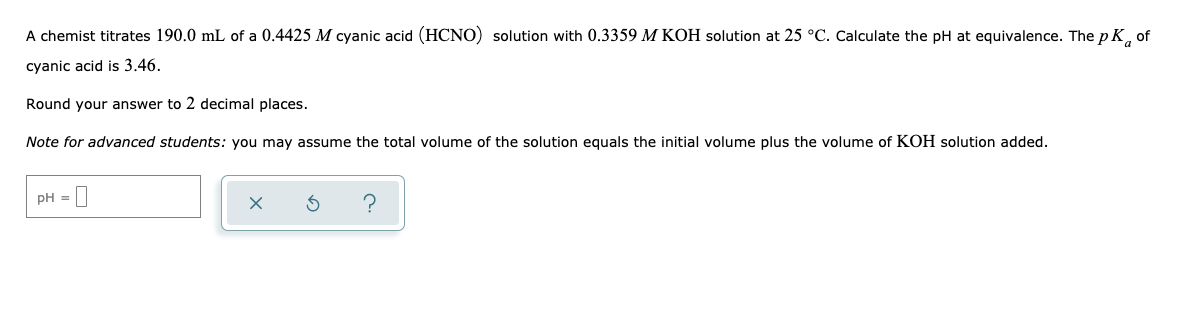A chemist titrates 190.0 mL of a 0.4425 M cyanic acid (HCNO) solution with 0.3359 M KOH solution at 25 °C. Calculate the pH at equivalence. The pK, of cyanic acid is 3.46. Round your answer to 2 decimal places. Note for advanced students: you may assume the total volume of the solution equals the initial volume plus the volume of KOH solution added. pH = D x 6 ? |

• ### An analytical chemist is titrating 54.4 ml of a 0.9400 M solution of acetic acid (HCH,CO,)...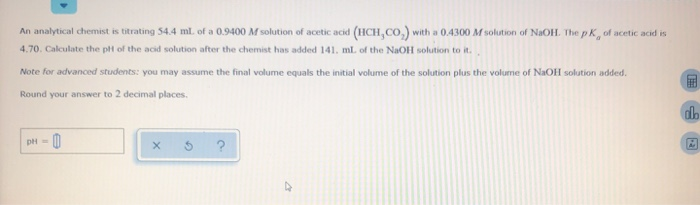An analytical chemist is titrating 54.4 ml of a 0.9400 M solution of acetic acid (HCH,CO,) with a 0.4300 M solution of NaOH. The pk of acetic acid is 4.70. Calculate the pit of the acid solution after the chemist has added 141 ml of the NaOH solution to it. Note for advanced students: you may assume the final volume equals the initial volume of the solution plus the volume of NaOH solution added. Round your answer to 2 decimal...

• ### An analytical chemist is titrating 69.7 mL of a 0.3900 M solution of acetic acid (HCH,...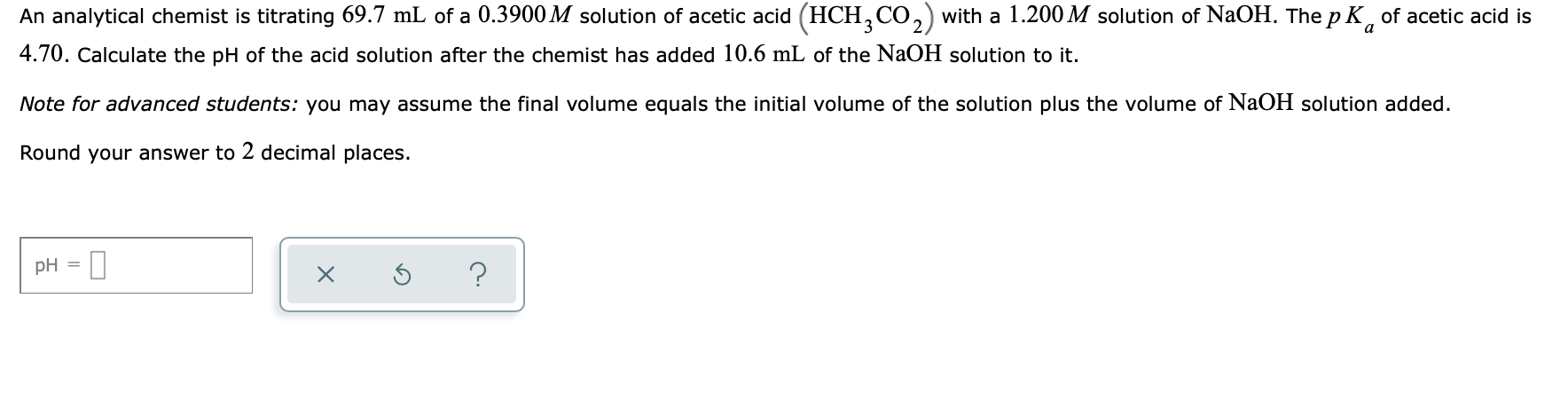An analytical chemist is titrating 69.7 mL of a 0.3900 M solution of acetic acid (HCH, CO,) with a 1.200 M solution of NaOH. The pK, of acetic acid is 4.70. Calculate the pH of the acid solution after the chemist has added 10.6 mL of the NaOH solution to it. Note for advanced students: you may assume the final volume equals the initial volume of the solution plus the volume of NaOH solution added. Round your answer to 2...

• ### An analytical chemist is titrating 182.1 mL. of a 0.4900 M solution of acetic acid (HCH,CO,)...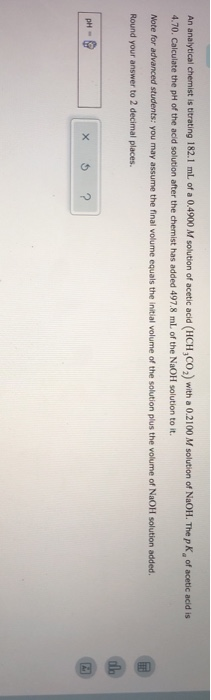An analytical chemist is titrating 182.1 mL. of a 0.4900 M solution of acetic acid (HCH,CO,) with a 0.2100 M solution of NaOH. The p K of acetic acid is 4.70. Calculate the pH of the acid solution after the chemist has added 497.8 ml of the NaOH solution to it. Note for advanced students: you may assume the final volume equals the initial volume of the solution plus the volume of NaOH solution added. Round your answer to 2...

• ### An analytical chemist is titrating 106,3 ml. of a 0.9500 M solution of acetic acid (HCH,CO,)...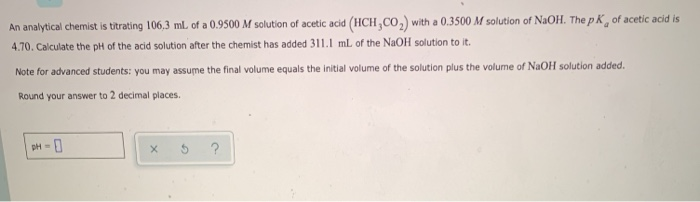An analytical chemist is titrating 106,3 ml. of a 0.9500 M solution of acetic acid (HCH,CO,) with a 0.3500 M solution of NaOH. The p K, of acetic acid is 4.70. Calculate the pH of the acid solution after the chemist has added 311.1 mL of the NaOH solution to it. Note for advanced students: you may assume the final volume equals the initial volume of the solution plus the volume of NaOH solution added. Round your answer to 2...

• ### An analytical chemist is titrating 72.8 ml. of a 0.6300 M solution of acetic acid (HCH,CO,) with a 0.6600 M solutio...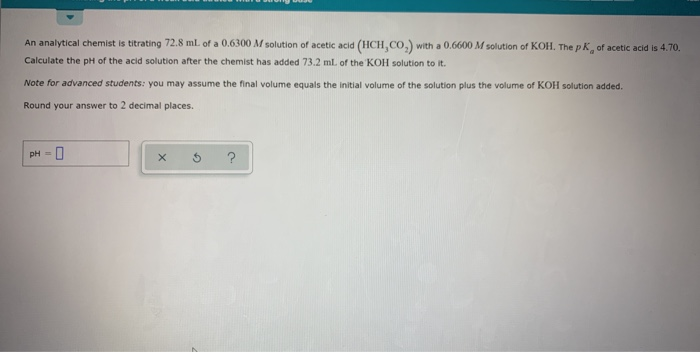An analytical chemist is titrating 72.8 ml. of a 0.6300 M solution of acetic acid (HCH,CO,) with a 0.6600 M solution of KOH. The pk, of acetic acid is 4.70. Calculate the pH of the acid solution after the chemist has added 73.2 ml of the KOH solution to it Note for advanced students: you may assume the final volume equals the initial volume of the solution plus the volume of KOH solution added. Round your answer to 2 decimal...

• ### A chemist titrates 200.0 mL of a 0.7681 M hydrocyanic acid (HCN) solution with 0.5271 M...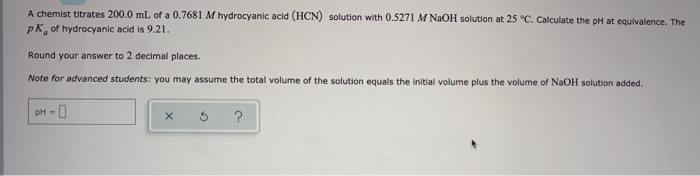A chemist titrates 200.0 mL of a 0.7681 M hydrocyanic acid (HCN) solution with 0.5271 M NaOH solution at 25 °C. Calculate the pH at equivalence. The pk of hydrocyanic acid is 9.21. Round your answer to 2 decimal places. Note for advanced students: you may assume the total volume of the solution equals the initial volume plus the volume of NaOH solution added. pH- х ?

• ### A chemist titrates 100.0 ml of a 0,6612 M hydrocyanic acid (HCN) solution with 0.8512 M NaOH solution at 25 c. Calc...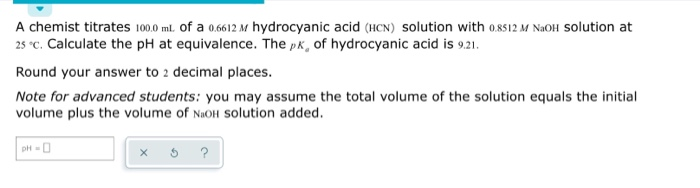A chemist titrates 100.0 ml of a 0,6612 M hydrocyanic acid (HCN) solution with 0.8512 M NaOH solution at 25 c. Calculate the pH at equivalence. The pk, of hydrocyanic acid is 9.21. Round your answer to 2 decimal places. Note for advanced students: you may assume the total volume of the solution equals the initial volume plus the volume of N.OH solution added. pH- x 5 ?

• ### A chemist titrates 160.0 mL of a 0.3337 M carbonic acid (H2CO3) solution with 0.4095 M...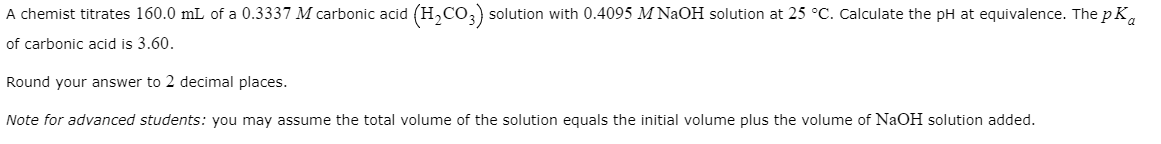A chemist titrates 160.0 mL of a 0.3337 M carbonic acid (H2CO3) solution with 0.4095 M NaOH solution at 25 °C. Calculate the pH at equivalence. The pK, of carbonic acid is 3.60. Round your answer to 2 decimal places. Note for advanced students: you may assume the total volume of the solution equals the initial volume plus the volume of NaOH solution added.

• ### A chemist titrates 90.0 mL of a 0.5705 M carbonic acid (H,CO,) solution with 0.6274 M...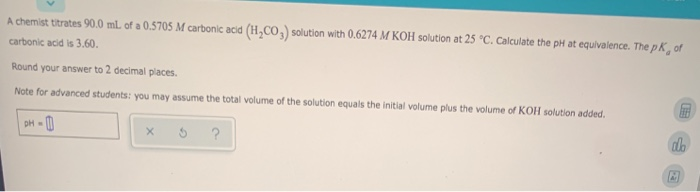A chemist titrates 90.0 mL of a 0.5705 M carbonic acid (H,CO,) solution with 0.6274 M KOH solution at 25 °C. Calculate the pH at equivalence. The pk of carbonic acid is 3.60. Round your answer to 2 decimal places. Note for advanced students: you may assume the total volume of the solution equals the initial volume plus the volume of KOH solution added. DH-